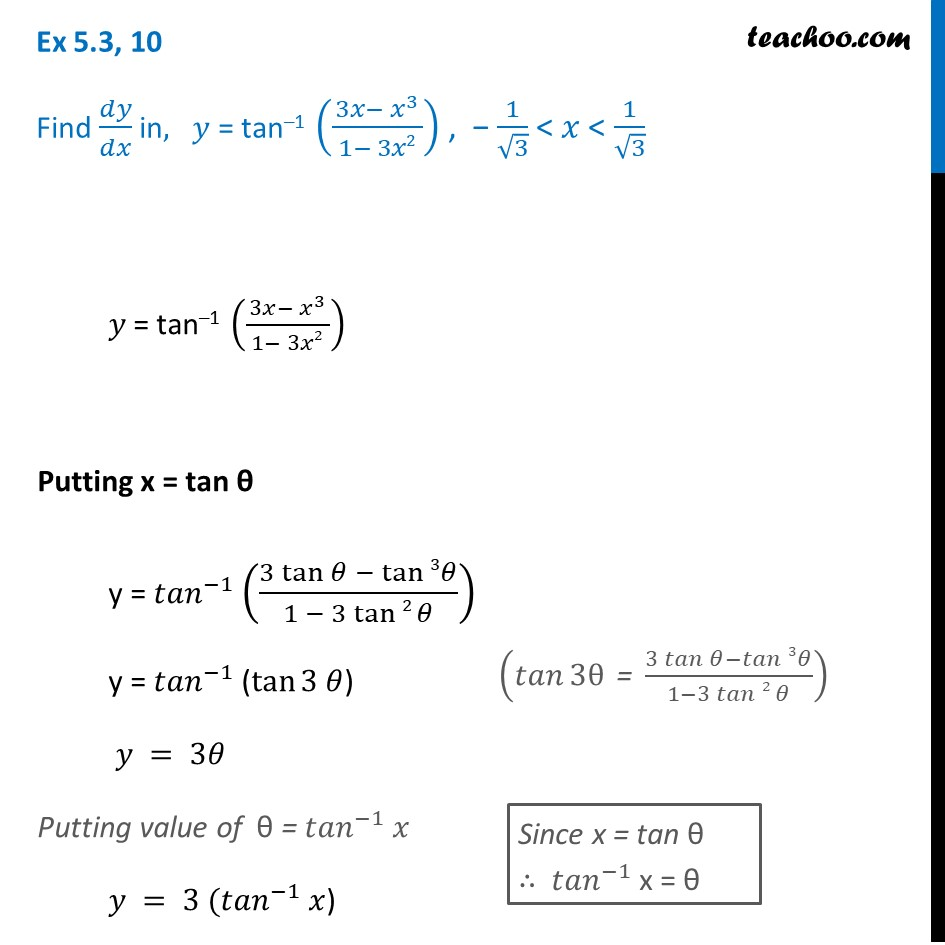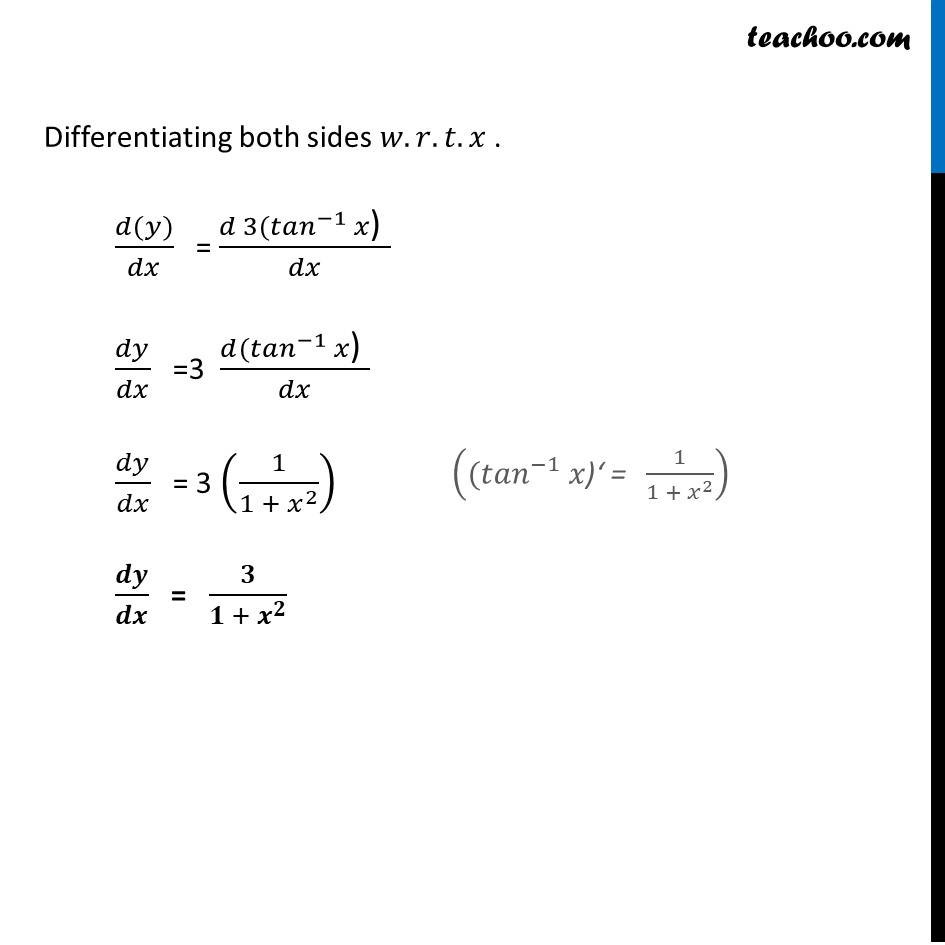Finding derivative of Inverse trigonometric functions

Chapter 5 Class 12 Continuity and Differentiability
Concept wiseIntroducing your new favourite teacher - Teachoo Black, at only ₹83 per month

### Transcript

Ex 5.3, 10 Find 𝑑𝑦/𝑑𝑥 in, 𝑦 = tan–1 ((3𝑥− 𝑥^3)/( 1− 3𝑥2 )) , − 1/√3 < 𝑥 < 1/√3 𝑦 = tan–1 ((3𝑥− 𝑥^3)/( 1− 3𝑥2 )) Putting x = tan θ y = 〖𝑡𝑎𝑛〗^(−1) ((3 tan⁡〖𝜃 − tan⁡3 𝜃〗)/(1 − 3 tan⁡2 𝜃)) y = 〖𝑡𝑎𝑛〗^(−1) (tan⁡〖3 𝜃〗) 𝑦 = 3𝜃 Putting value of θ = 〖𝑡𝑎𝑛〗^(−1) 𝑥 𝑦 = 3〖 (𝑡𝑎𝑛〗^(−1) 𝑥) (𝑡𝑎𝑛⁡3θ " = " (3 𝑡𝑎𝑛⁡〖𝜃−𝑡𝑎𝑛⁡3 𝜃〗)/(1−3 𝑡𝑎𝑛⁡2 𝜃)) Since x = tan θ ∴ 〖𝑡𝑎𝑛〗^(−1) x = θ Differentiating both sides 𝑤.𝑟.𝑡.𝑥 . (𝑑(𝑦))/𝑑𝑥 = (𝑑 3〖(𝑡𝑎𝑛〗^(−1) 𝑥") " )/𝑑𝑥 𝑑𝑦/𝑑𝑥 =3 (𝑑〖(𝑡𝑎𝑛〗^(−1) 𝑥") " )/𝑑𝑥 𝑑𝑦/𝑑𝑥 = 3 (1/(1 + 𝑥^2 )) 𝒅𝒚/𝒅𝒙 = 𝟑/(𝟏 +〖 𝒙〗^𝟐 ) ((〖𝑡𝑎𝑛〗^(−1) 𝑥")‘ = " 1/(1 + 𝑥^2 ))